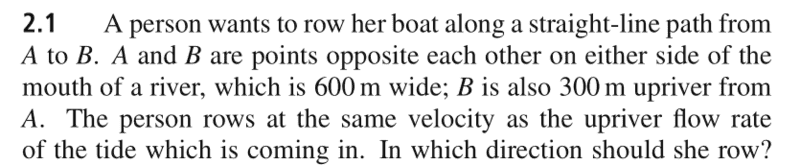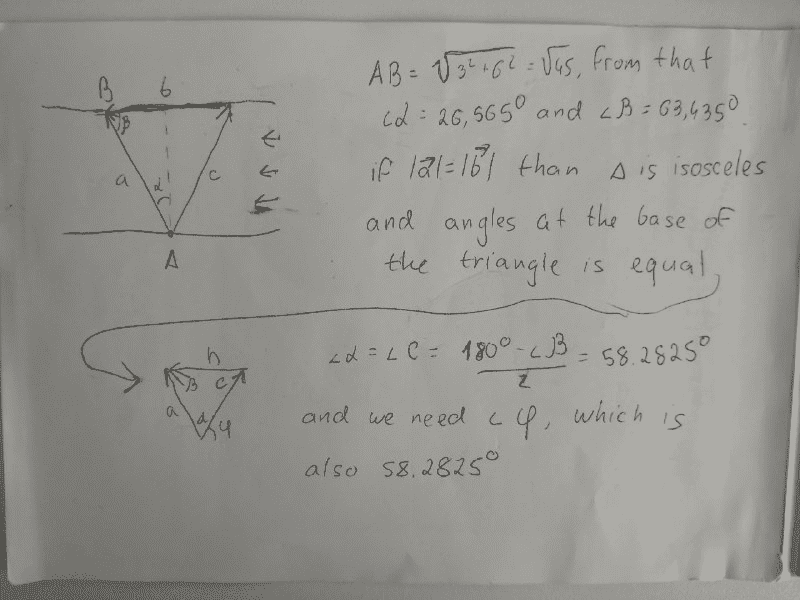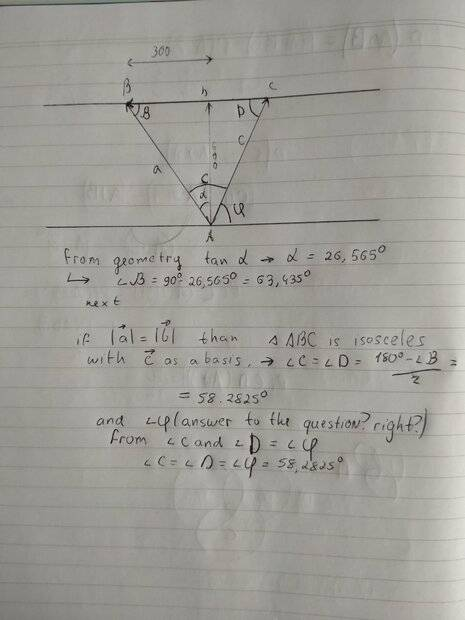• evo13
In summary, the question is asking for the direction of the boat relative to the water. The answer is given by vector addition.f

#### evo13

Homework Statement
Introduction physics
Relevant EquationsHello, guys. Interesting riddle here.
I have no idea how to solve it. Tried different methods, but point is answer is always wrong,
exact answer Downriver, at an angle of 53.13(degree) to the bank.
That exercise is from
"Pohl’s Introduction to Physics"

The basic idea is that the velocity of the boat relative to the banks is the vector sum of the velocity of the river relative to the banks and the velocity of the boat relative to the river.

Yes, and i can't get a right answer. Basic idea is that velocity of boat and river is the same magnitude, at least as i understand it

Yes, and i can't get a right answer. Basic idea is that velocity of boat and river is the same magnitude, at least as i understand it

PS The given answer of 53.13 degress is correct.Sorry for the sloppiness, this is just one of the solutions I tried

You know that the river is ##600m## wide and it's ##300m## along the bank from ##A## to ##B##. The angle ##\alpha## can be calculated from this geometry.

I.e. ##\tan \alpha = 1/2##

You should get the answer from that.

You know that the river is ##600m## wide and it's ##300m## along the bank from ##A## to ##B##. The angle ##\alpha## can be calculated from this geometry.

I.e. ##\tan \alpha = 1/2##

You should get the answer from that.

Yep, i did that. ∠α is 26,565 degrees
AB = sqrt(45) is not relevant, sorry.

Yep, i did that. ∠α is 26,565 degrees
AB = sqrt(45) is not relevant, sorry.
That gives you the direction relative to the bank. The question wants the direction relative to the water, which means you have to do vector addition.

Sorry but vector addition is second part of my solving attempt, below horizontal line.
I don't understand what else i can do

PS I don't see how you got 58.3 degrees. You should have got 63.4 degrees upstream as the direction relative to the bank. But, that is independent of the velocity of the river.

lets try another explanation, more clear i hopeOkay, I see what you've done. Why would ##a = b##?

Note that your diagram is based on the reference frame of the banks. It's difficult to indicate the velocity of the boat relative to the river on that diagram.

Vector addition is the way to go!

if not a = b, than c = b. Right?
I tried this also

if not a = b, than c = b. Right?
I tried this also
Neither. Your fundamental problem is that ##c## is from the river's frame of reference and ##a## is from the banks frame of reference. You are mixing vectors from two reference frames in one diagram.

Even if these equations were to hold, they can't be shown in a single diagram.